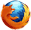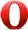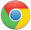# JavaScript atan2() 方法JavaScript Math 对象

## 实例 1 - atan2(y,x)

Math.atan2(8,4);

1.1071487177940904

## 定义和用法

atan2() 返回从原点(0,0) 到 (x,y) 点的线段与 x 轴正方向之间的平面角度(弧度值)，也就是 Math.atan2(y,x)

## 浏览器支持Math.atan2(y,x)

y 必须。一个数字代表Y坐标
x 必须。 一个数字代表x坐标

## 返回值

Number x 的反正切值。返回一个 -PI 到 PI 之间的数值。表示点 (x, y) 对应的偏移角度。这是一个逆时针角度，以弧度为单位，正X轴和点 (x, y) 与原点连线 之间。注意此函数接受的参数：先传递 y 坐标，然后是 x 坐标。

## 技术细节

 JavaScript 版本： 1

## 实例

Math.atan2(90, 15) // 1.4056476493802699
Math.atan2(15, 90) // 0.16514867741462683

Math.atan2( ±0, -0 )               // ±PI.
Math.atan2( ±0, +0 )               // ±0.
Math.atan2( ±0, -x )               // ±PI for x > 0.
Math.atan2( ±0, x )                // ±0 for x > 0.
Math.atan2( -y, ±0 )               // -PI/2 for y > 0.
Math.atan2( y, ±0 )                // PI/2 for y > 0.
Math.atan2( ±y, -Infinity )        // ±PI for finite y > 0.
Math.atan2( ±y, +Infinity )        // ±0 for finite y > 0.
Math.atan2( ±Infinity, x )         // ±PI/2 for finite x.
Math.atan2( ±Infinity, -Infinity ) // ±3*PI/4.
Math.atan2( ±Infinity, +Infinity ) // ±PI/4.JavaScript Math 对象